# Exponential and Logarithmic Models

While we have explored some basic applications of exponential and logarithmic functions, in this section we explore some important applications in more depth.

More complex exponential equations can often be solved in more than one way. In the following example, we will solve the same problem in two ways – one using logarithm properties, and the other using exponential properties.

Example 4.3.1a

In 2008, the population of Kenya was approximately 38.8 million, and was growing by 2.64% each year, while the population of Sudan was approximately 41.3 million and growing by 2.24% each year. If these trends continue, when will the population of Kenya match that of Sudan?

We start by writing an equation for each population in terms of t, the number of years after 2008.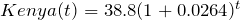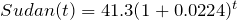To find when the populations will be equal, we can set the equations equal: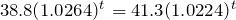For our first approach, we take the log of both sides of the equation: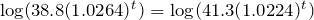Utilizing the sum property of logs, we can rewrite each side: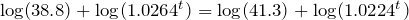Then utilizing the exponent property, we can pull the variables out of the exponent: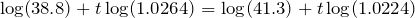Moving all the terms involving t to one side of the equation and the rest of the terms to the other side: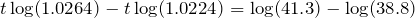Factoring out the t on the left: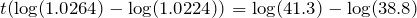Dividing to solve for t: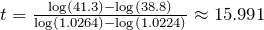years until the populations will be equal.

Example 4.3.1b

Solve the problem above by rewriting before taking the log.

Starting at the equation:

38.8(1.0264)t = 41.3(1.0224)t

Divide to move the exponential terms to one side of the equation and the constants to the other side: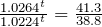Using exponent rules to group on the left: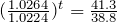Taking the log of both sides: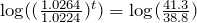Utilizing the exponent property on the left: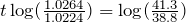Dividing gives: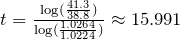years

While the answer does not immediately appear identical to that produced using the previous method, note that by using the difference property of logs, the answer could be rewritten: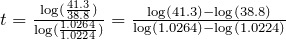While both methods work equally well, it often requires fewer steps to utilize algebra before taking logs, rather than relying solely on log properties.

In an earlier section, we discussed radioactive decay – the idea that radioactive isotopes change over time. One of the common terms associated with radioactive decay is half-life.

Half Life: The half-life of a radioactive isotope is the time it takes for half the substance to decay.

Given the basic exponential growth/decay equation h(t) = abt , half-life can be found by solving for when half the original amount remains; by solving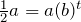, or more simply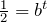. Notice how the initial amount is irrelevant when solving for half-life.

Example 4.3.2

Bismuth-210 is an isotope that decays by about 13% each day. What is the half-life of Bismuth-210?

We were not given a starting quantity, so we could either make up a value or use an unknown constant to represent the starting amount. To show that starting quantity does not affect the result, let us denote the initial quantity by the constant a. Then the decay of Bismuth-210 can be described by the equation Q(d) = a(0.87)d .

To find the half-life, we want to determine when the remaining quantity is half the original: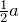. Solving: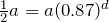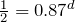Dividing by a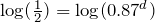Taking the log of both sides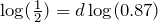Use the exponent property of logs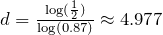days Divide to solve for d

This tells us that the half-life of Bismuth-210 is approximately 5 days.

Example 4.3.3

Cesium-137 has a half-life of about 30 years. If you begin with 200 mg of cesium-137, how much will remain after 30 years? 60 years? 90 years?

Since the half-life is 30 years, after 30 years, half the original amount, 100 mg, will remain.

After 60 years, another 30 years have passed, so during that second 30 years, another half of the substance will decay, leaving 50 mg.

After 90 years, another 30 years have passed, so another half of the substance will decay, leaving 25 mg.

Example 4.3.4

Carbon-14 is a radioactive isotope that is present in organic materials, and is commonly used for dating historical artifacts. Carbon-14 has a half-life of 5730 years. If a bone fragment is found that contains 20% of its original carbon-14, how old is the bone?

To find how old the bone is, we first will need to find an equation for the decay of the carbon-14. We could either use a continuous or annual decay formula, but opt to use the continuous decay formula since it is more common in scientific texts. The half life tells us that after 5730 years, half the original substance remains. Solving for the rate: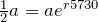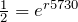Dividing by a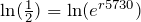Taking the natural log of both sides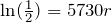Use the inverse property of logs on the right side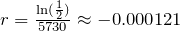Dividing by 5730

Now we know the decay will follow the equation Q(t) = ae-0.000121t . To find how old the bone fragment is that contains 20% of the original amount, we solve for t so that Q(t) = 0.20a.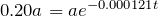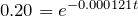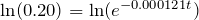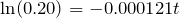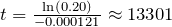years.

Therefore, the bone fragment is about 13,300 years old.

# Doubling Time

For decaying quantities, we asked how long it takes for half the substance to decay. For growing quantities, we might ask how long it takes for the quantity to double.

Doubling Time: The doubling time of a growing quantity is the time it takes for the quantity to double.

Given the basic exponential growth equation h(t) = abt , doubling time can be found by solving for when the original quantity has doubled; by solving 2a = a(b)x , or more simply 2 = bx. Again notice how the initial amount is irrelevant when solving for doubling time.

Example 4.3.5

If you invest money at 8% compounded quarterly, how long will it take your money to double?

Using the compound interest equation, we can write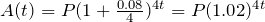.

To find the doubling time, we look for the time until we have twice the original amount, so when A(t) = 2P. Notice we don’t need to know how much was invested.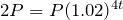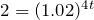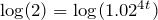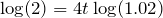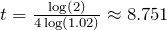years.

It will take about 8.75 years for the investment double in value.

Example 4.3.6

Use of a new social networking website has been growing exponentially, with the number of new members doubling every 5 months. If the site currently has 120,000 users and this trend continues, how many users will the site have in 1 year?

We can use the doubling time to find a function that models the number of site users, and then use that equation to answer the question. While we could use an arbitrary a as we have before for the initial amount, in this case, we know the initial amount was 120,000.

If we use a continuous growth equation, it would look like N(t) = 120ert , measured in thousands of users after t months. Based on the doubling time, there would be 240 thousand users after 5 months. This allows us to solve for the continuous growth rate: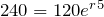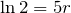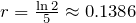Now that we have an equation, N(t) = 120e0.1386t , we can predict the number of users after 12 months:

N(12) = 120e0.1386(12) = 633.140 thousand users.

So after 1 year, we would expect the site to have around 633,140 users.

# Practice questions

1. Tank A contains 10 litres of water, and 35% of the water evaporates each week. Tank B contains 30 litres of water, and 50% of the water evaporates each week. In how many weeks will the tanks contain the same amount of water?

2. In 2020, the population of Canada was approximately 37,742,154, with an annual growth rate of 0.89%. In the same year, the population of Saudi Arabia was 34,813,871, with an annual growth rate of 1.59%. Assuming these trends continue, when would the populations of these two countries be equal?

3. Recall that Cesium-137 has a half-life of about 30 years. If you begin with 200 mg of Cesium-137, how long will it take until only 1 milligram remains?

4. Plutonium-239, a product of nuclear explosion, has a half-life of 24,000 years. What percent of plutonium-239 would remain after:

a. 100 years

b. 1000 years

c. 10,000 years

5. If tuition at a university is increasing by 6.6% each year, how many years will it take for tuition to double?

6. Suppose a new strain of influenza has emerged and the number of cases worldwide is doubling exponentially every 7 months. What is the continuous growth rate (r)?